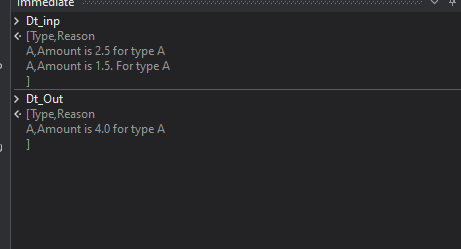need to add same data in datatable

Eg,:

Type. Reason

A. Amount is 2.5 for type A
A Amount is 1.5. For type A

Since in datatable boththe type is same.i need to add the value in reason my output should be

Type. Reason
A. Amount is 4.0 for type A

Checkout this expression

``````(From row In Dt_inp.AsEnumerable
Group row By sc = row("Type").ToString()
Into grp = Group
Let total= grp.sum(Function (x) Convert.ToDouble(System.Text.RegularExpressions.Regex.Match(x("Reason").ToString,"\d+\.\d+").ToString))
Let type=grp(0)("Type").ToString
Let result = New Object() {type, "Amount is "+CDec(total).ToString("F1")+" for type "+type}
``````Hope this helps!

Regards
Sudharsan

Find the sample xaml file for your reference @sruthesanju

Main.xaml (8.9 KB)

Regards
Sudharsan